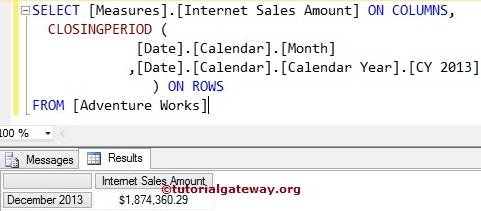# MDX ClosingPeriod Function

The MDX ClosingPeriod Function will return the Last Sibling member belongs to the specified member or at a specified level.

## MDX ClosingPeriod Function Syntax

The basic syntax of the ClosingPeriod in Multidimensional Expression is as shown below:

`CLOSINGPERIOD (Level_Expression, Member_Expression)`
• Member_Expression: Any Multidimensional Expression that returns valid Member.
• Level_Expression: Please specify the level you want to navigate.

For this ClosingPeriod function in MDX example, We are going to use the below show data. The following screenshot shows the List of Calender Years inside the Date Dimension

## MDX ClosingPeriod Function Example

In this example, we are going to find the Last year present in the Calendar Year using the MDX ClosingPeriod function.

```SELECT
[Measures].[Internet Sales Amount] ON COLUMNS,
CLOSINGPERIOD ([Date].[Calendar].[Calendar Year]) ON ROWS

In the above MDX Query, We used [Internet Sales amount] on the columns

`[Measures].[Internet Sales Amount] ON COLUMNS`

Below line of code will return the last sibling member present in the Calendar Year from the Date Dimension, Which is the Year 2014.

`CLOSINGPERIOD ([Date].[Calendar].[Calendar Year])`

## MDX ClosingPeriod Function Example 2

In this example, we are going to find the Last Month present in the Calendar Year 2013 using ClosingPeriod function and calculate the Internet Sales Amount of that.

```SELECT
[Measures].[Internet Sales Amount] ON COLUMNS,
CLOSINGPERIOD (
[Date].[Calendar].[Month]
,[Date].[Calendar].[Calendar Year].[CY 2013]
) ON ROWS
```## Range Using MDX ClosingPeriod Example 1

In this example, we show you how to find the Range using the ClosingPeriod function. The following MDX query will return the Internet Sales amount from Calendar Year 2010 to 2014

```SELECT
[Measures].[Internet Sales Amount] ON COLUMNS,
[Date].[Calendar].[Calendar Year].[CY 2010]:
CLOSINGPERIOD ([Date].[Calendar].[Calendar Year]) ON ROWS

In the above MDX Query, We used [Internet Sales amount] on the columns

`[Measures].[Internet Sales Amount] ON COLUMNS`

The below line of code will return the last sibling member present in the Calendar Year from the Date Dimension, Which is 2014.

`CLOSINGPERIOD ([Date].[Calendar].[Calendar Year])`

It means We used the range operator between 2010 and 2014 Year. So, the output will display the Internet Sales amount of all the Calender Years present in the Date dimension.

```[Date].[Calendar].[Calendar Year].[CY 2010] -- 2010

CLOSINGPERIOD ([Date].[Calendar].[Calendar Year]): -- 2014```

## Range Using MDX ClosingPeriod Example 2

In this example, we show how to find the Range using the ClosingPeriod function. The following query will return the Internet Sales amount from May to December Month in Calendar Year 2013

```SELECT
[Measures].[Internet Sales Amount] ON COLUMNS,
[Date].[Calendar].[Month].[May 2013]:
CLOSINGPERIOD (
[Date].[Calendar].[Month]
,[Date].[Calendar].[Calendar Year].[CY 2013]
) ON ROWS